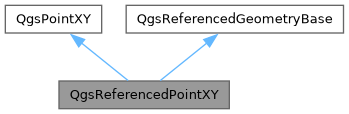QGIS API Documentation 3.35.0-Master (728e5967df5)
Searching...
No Matches
QgsReferencedPointXY Class Reference

A QgsPointXY with associated coordinate reference system. More...

`#include <qgsreferencedgeometry.h>`

Inheritance diagram for QgsReferencedPointXY:[legend]

## Public Member Functions

QgsReferencedPointXY ()=default
Constructor for QgsReferencedPointXY.

QgsReferencedPointXY (const QgsPointXY &point, const QgsCoordinateReferenceSystem &crs)
Constructor for QgsReferencedPointXY, with the specified initial point and crs.

operator QVariant () const
Allows direct construction of QVariants from point.

bool operator!= (const QgsReferencedPointXY &other)

bool operator== (const QgsReferencedPointXY &other)Public Member Functions inherited from QgsPointXY
QgsPointXY ()=default
Default constructor.

QgsPointXY (const QgsPoint &point)
Create a new point.

QgsPointXY (const QgsPointXY &p)
Create a point from another point.

QgsPointXY (double x, double y)
Create a point from x,y coordinates.

QgsPointXY (QPoint point)
Create a point from a QPoint.

QgsPointXY (QPointF point)
Create a point from a QPointF.

~QgsPointXY ()=default

QString asWkt () const
Returns the well known text representation for the point (e.g.

double azimuth (const QgsPointXY &other) const
Calculates azimuth between this point and other one (clockwise in degree, starting from north)

bool compare (const QgsPointXY &other, double epsilon=4 *std::numeric_limits< double >::epsilon()) const
Compares this point with another point with a fuzzy tolerance.

double distance (const QgsPointXY &other) const
Returns the distance between this point and another point.

double distance (double x, double y) const
Returns the distance between this point and a specified x, y coordinate.

bool isEmpty () const
Returns `true` if the geometry is empty.

void multiply (double scalar)
Multiply x and y by the given value.

operator QVariant () const
Allows direct construction of QVariants from points.

bool operator!= (const QgsPointXY &other) const
Inequality operator.

QgsPointXY operator* (double scalar) const
Multiplies the coordinates in this point by a scalar quantity.

QgsPointXYoperator*= (double scalar)
Multiplies the coordinates in this point by a scalar quantity in place.

QgsPointXY operator+ (QgsVector v) const
Adds a vector to this point.

QgsPointXYoperator+= (QgsVector v)
Adds a vector to this point in place.

QgsVector operator- (const QgsPointXY &p) const
Calculates the vector obtained by subtracting a point from this point.

QgsPointXY operator- (QgsVector v) const
Subtracts a vector from this point.

QgsPointXYoperator-= (QgsVector v)
Subtracts a vector from this point in place.

QgsPointXY operator/ (double scalar) const
Divides the coordinates in this point by a scalar quantity.

QgsPointXYoperator/= (double scalar)
Divides the coordinates in this point by a scalar quantity in place.

QgsPointXYoperator= (const QgsPointXY &other)
Assignment.

bool operator== (const QgsPointXY &other)
equality operator

QgsPointXY project (double distance, double bearing) const
Returns a new point which corresponds to this point projected by a specified distance in a specified bearing.

void set (double x, double y)
Sets the x and y value of the point.

void setX (double x)
Sets the x value of the point.

void setY (double y)
Sets the y value of the point.

double sqrDist (const QgsPointXY &other) const
Returns the squared distance between this point another point.

double sqrDist (double x, double y) const
Returns the squared distance between this point a specified x, y coordinate.

double sqrDistToSegment (double x1, double y1, double x2, double y2, QgsPointXY &minDistPoint, double epsilon=DEFAULT_SEGMENT_EPSILON) const
Returns the minimum distance between this point and a segment.

QPointF toQPointF () const
Converts a point to a QPointF.

QString toString (int precision=-1) const
Returns a string representation of the point (x, y) with a preset precision.

double x () const
Gets the x value of the point.

double y () const
Gets the y value of the point.Public Member Functions inherited from QgsReferencedGeometryBase
QgsReferencedGeometryBase (const QgsCoordinateReferenceSystem &crs=QgsCoordinateReferenceSystem())
Constructor for QgsReferencedGeometryBase, with the specified crs.

QgsCoordinateReferenceSystem crs () const
Returns the associated coordinate reference system, or an invalid CRS if no reference system is set.

void setCrs (const QgsCoordinateReferenceSystem &crs)
Sets the associated crs.Properties inherited from QgsPointXY
double x

double y

## Detailed Description

A QgsPointXY with associated coordinate reference system.

Since
QGIS 3.0

Definition at line 114 of file qgsreferencedgeometry.h.

## ◆ QgsReferencedPointXY() [1/2]

 QgsReferencedPointXY::QgsReferencedPointXY ( const QgsPointXY & point, const QgsCoordinateReferenceSystem & crs )

Constructor for QgsReferencedPointXY, with the specified initial point and crs.

Definition at line 39 of file qgsreferencedgeometry.cpp.

## ◆ QgsReferencedPointXY() [2/2]

 QgsReferencedPointXY::QgsReferencedPointXY ( )
default

Constructor for QgsReferencedPointXY.

## ◆ operator QVariant()

 QgsReferencedPointXY::operator QVariant ( ) const
inline

Allows direct construction of QVariants from point.

Definition at line 130 of file qgsreferencedgeometry.h.

## ◆ operator!=()

 bool QgsReferencedPointXY::operator!= ( const QgsReferencedPointXY & other )

Definition at line 49 of file qgsreferencedgeometry.cpp.

## ◆ operator==()

 bool QgsReferencedPointXY::operator== ( const QgsReferencedPointXY & other )

Definition at line 44 of file qgsreferencedgeometry.cpp.

The documentation for this class was generated from the following files: